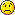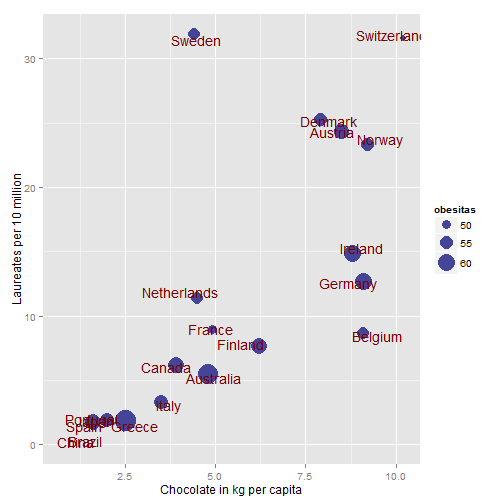# Chocolate and nobel prize – a true story?

December 22, 2012
By

Want to share your content on R-bloggers? click here if you have a blog, or here if you don't.Few of us can resist chocolate, but the real question is: should we even try to resist it? The image is CC by Tasumi1968.
As a dark chocolate addict I was relieved to see Messerli’s ecological study on chocolate consumption and the relation to nobel prizes. By scraping various on-line sources he made a robust case for increased chocolate consumption correlating well to number of nobel prizes of that country. This in addition that it might have positive impact on hypertension, is strong enough evidence for me to avoid changing my habits, at least over Christmas## Tutorial: Scraping the chocolate data with R

Inspired by Messerli’s article I decided to look into how to repeat the analysis in R. First we get nobel prizes per country by reading the table using readHTMLTable() from the XML package. After that we do some cleaning to the dataset.
?View Code RSPLUS

 ```1 2 3 4 5 6 7 8 9 10 11 12 13 14 15 16 17 18 19 20 21 22 23 24 ``` ```library(XML) theurl <- "http://en.wikipedia.org/wiki/List_of_countries_by_Nobel_laureates_per_capita" tables <- readHTMLTable(theurl)   nobel_prizes <- tables[] # Clean column names colnames(nobel_prizes) <- gsub(" ", "_", gsub("(/|\\[[0-9]+\\])", "", gsub("\n", " ", colnames(nobel_prizes)) ) )   # Delete those that aren't countries and thus lack rank nobel_prizes\$Rank <- as.numeric(as.character(nobel_prizes\$Rank)) nobel_prizes <- subset(nobel_prizes, is.na(Rank) == FALSE)   # Clean the country names nobel_prizes\$Country <- gsub("[^a-zA-Z ]", "", nobel_prizes\$Country)   # Clean the loriates variable nobel_prizes\$Laureates_10_million <- as.numeric(as.character(nobel_prizes\$Laureates_10_million))```

### Chocolate data

First occurence we set by hand since it’s only one as specified by the article.
?View Code RSPLUS

 ```1 2 3 ``` ```nobel_prizes\$Chocolate_consumption <- NA # http://www.chocosuisse.ch/web/chocosuisse/en/documentation/faq.html nobel_prizes\$Chocolate_consumption[nobel_prizes\$Country == "Switzerland"] <- 11.9```
The next part is slightly trickier since we need to translate german country names to match the nobel prize data.
?View Code RSPLUS

 ```1 2 3 4 5 6 7 8 9 10 ``` ```# Translation from German to English theurl <- "http://german.about.com/library/blnation_index.htm"   tables <- readHTMLTable(theurl)   translate_german <- tables[] translate_german <- translate_german[3:NROW(translate_german), 1:2] colnames(translate_german) <- c("English", "German") translate_german\$German <- gsub("([ ]+(f|pl)\\.\$|\\([[:alnum:] -]+\\))", "", translate_german\$German)```
Now lets go for the actual data
?View Code RSPLUS

 ```1 2 3 4 5 6 7 8 9 10 11 12 13 14 15 16 17 18 19 20 21 22 23 24 25 26 27 28 29 30 31 32 33 34 35 36 37 38 39 40 41 42 43 44 45 46 47 48 49 50 51 52 53 54 ``` ```# Get the consumption from a German list theurl <- "http://www.theobroma-cacao.de/wissen/wirtschaft/international/konsum"   tables <- readHTMLTable(theurl)   german_chocolate_data <- tables[][2:NROW(tables[]), ] names(german_chocolate_data) <- c("Country", "Chocolate_consumption") german_chocolate_data\$Country <- gsub("([ ]+f\\.\$|\\([[:alnum:] -]+\\))", "", german_chocolate_data\$Country)   library(sqldf)   sql <- paste0("SELECT gc.*, tg.English as Country_en", " FROM german_chocolate_data AS gc", " LEFT JOIN translate_german AS tg", " ON gc.Country = tg.German", " OR gc.Country = tg.English") german_chocolate_data <- sqldf(sql)   german_chocolate_data\$Country <- ifelse(is.na(german_chocolate_data\$Country_en), german_chocolate_data\$Country, german_chocolate_data\$Country_en)   german_chocolate_data\$Country_en <- NULL german_chocolate_data\$Chocolate_consumption_tr <- NA for (i in 1:NROW(german_chocolate_data)) { number <- as.character(german_chocolate_data\$Chocolate_consumption[i]) if (length(number) > 0) { m <- regexpr("^([0-9]+,[0-9]+)", number) if (m > 0) { german_chocolate_data\$Chocolate_consumption_tr[i] <- as.numeric( sub(",", ".", regmatches(number, m)) ) } else { m <- regexpr("\\(([0-9]+,[0-9]+)", number)   if (m > 0) german_chocolate_data\$Chocolate_consumption_tr[i] <- as.numeric( sub("\\(", "", sub(",", ".", regmatches(number, m)) ) ) } } }   sql <- paste0("SELECT np.*, gp.Chocolate_consumption_tr AS choc", " FROM nobel_prizes AS np", " LEFT JOIN german_chocolate_data AS gp", " ON gp.Country = np.Country") nobel_prizes <- sqldf(sql)```
Unfortuntately the the Caobisco PDF can’t be found. Although it might be for the best since PDF data is hard to withdraw. One option could be to use the PDF to Word converter and hope for the best. It’s actually a quite impressive tool.
For our analysis we now have 20 countries with chocolate data. Lets plot it:
?View Code RSPLUS

 ```1 2 3 4 5 ``` ```library(ggplot2) ggplot(data = subset(nobel_prizes, is.na(choc) == FALSE), aes(x = choc, y = Laureates_10_million)) + ylab("Laureates per 10 million") + xlab("Chocolate in kg per capita") + geom_point(size = 4, colour = "#444499") + geom_text(aes(label = Country), size = 5, position = position_jitter(width = 0.5, height = 0.5), colour = "#990000")```### BMI – adding something new to the dataset

Now just to add some more fun to the data, lets look at obesity. I’ve found a simple table with male obesity available for scraping after a quick google search (yes I know, it’s only men, if you know a better table please post a comment and I’ll change it).
?View Code RSPLUS

 ```1 2 3 4 5 6 7 8 9 10 11 12 13 14 15 16 17 18 19 20 21 22 ``` ```# Percentage of males with a BMI > 25 kg/m2 tables <- readHTMLTable("http://www.oecd-ilibrary.org/sites/ovob-ml-table-2012-2-en/index.html;jsessionid=18wcxgabwn3ou.x-oecd-live-02?contentType=/ns/KeyTable,/ns/StatisticalPublication&itemId=/content/table/20758480-table16&containerItemId=/content/tablecollection/20758480&accessItemIds=&mimeType=text/html")   ob <- tables[] ob[, 2] <- as.character(ob[, 2]) ob <- apply(ob, FUN = as.character, MARGIN = 2) ob <- ob[, 2:NCOL(ob)] ob <- ob[4:NROW(ob), ]   last_obesitas <- apply(apply(ob[, 2:NCOL(ob)], FUN = as.numeric, MARGIN = 2), MARGIN = 1, FUN = function(x) { if (any(is.na(x) == FALSE)) return(x[max(which(is.na(x) == FALSE))]) else return(NA) })   ob <- data.frame(Country = ob[, 1], last_obesitas = last_obesitas) ob <- subset(ob, is.na(last_obesitas) == FALSE)   sql <- paste0("SELECT np.*, ob.last_obesitas AS obesitas", " FROM nobel_prizes AS np", " LEFT JOIN ob", " ON ob.Country = np.Country") nobel_prizes <- sqldf(sql)```
Now lets add it to our amazing plot:
?View Code RSPLUS

 ```1 2 3 4 5 ``` ```ggplot(data = subset(nobel_prizes, is.na(choc) == FALSE), aes(x = choc, y = Laureates_10_million)) + ylab("Laureates per 10 million") + xlab("Chocolate in kg per capita") + geom_point(aes(size = obesitas), colour = "#444499") + scale_size(range = c(3, 10)) + geom_text(aes(label = Country), size = 5, position = position_jitter(width = 0.5, height = 0.5), colour = "#770000")```A ggplot with the chocolate consumption and nobel lauriates with the obesity as size of the dots. It would be fun to add the country flags as in the original article. The names tend to get a little crowded, but I haven’t figured that one out yet… Ideally it would be something like the bus svg image in Murrel & Ly’s article in the R journal

### The model

Now if you want to compare our results to the original article you can find the model output below.
?View Code RSPLUS

 ```1 2 ``` ```fit <- lm(Laureates_10_million ~ choc, data = nobel_prizes) summary(fit)```
?View Code RSPLUS

 ```1 2 3 4 5 6 7 8 9 10 11 12 13 14 15 16 17 18 ``` ```## Call: ## lm(formula = Laureates_10_million ~ choc, data = nobel_prizes) ## ## Residuals: ## Min 1Q Median 3Q Max ## -11.94 -3.87 -1.28 2.16 22.77 ## ## Coefficients: ## Estimate Std. Error t value Pr(>|t|) ## (Intercept) -1.657 3.283 -0.50 0.61995 ## choc 2.442 0.541 4.51 0.00027 *** ## --- ## Signif. codes: 0 '***' 0.001 '**' 0.01 '*' 0.05 '.' 0.1 ' ' 1 ## ## Residual standard error: 7.45 on 18 degrees of freedom ## (51 observations deleted due to missingness) ## Multiple R-squared: 0.531, Adjusted R-squared: 0.505 ## F-statistic: 20.4 on 1 and 18 DF, p-value: 0.000269```

R-bloggers.com offers daily e-mail updates about R news and tutorials about learning R and many other topics. Click here if you're looking to post or find an R/data-science job.
Want to share your content on R-bloggers? click here if you have a blog, or here if you don't.